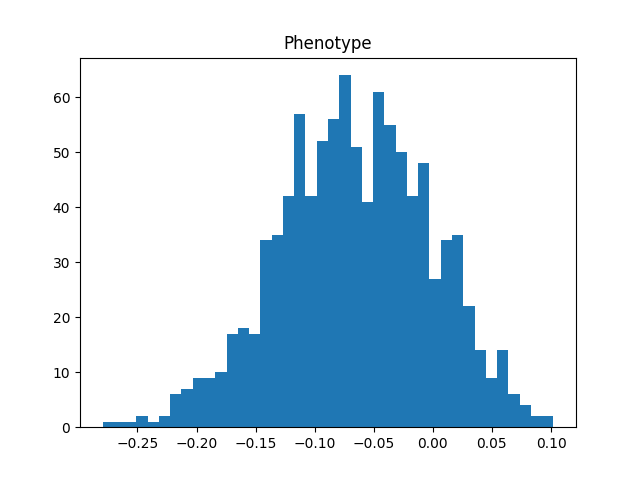# Ts-trait: new software for simulating quantitative traits (phenotypes)Often we want to map a genotype to a phenotype. A new `tskit`-based simulator leverages the succinct tree sequence format to do so efficiently for large numbers of genomes.

The new software, tstrait, takes an existing tree sequence containing mutations, and simulates a quantitative phenotypic trait whose value is partially determined by a selected set of causal mutations in the tree sequence.

This is an initial 0.0.1 release.

## Example code

``````import tstrait
import msprime
import matplotlib.pyplot as plt

anc_params = dict(recombination_rate=1e-8, sequence_length=1e5, population_size=10_000)

# Simulate a tree sequence of 1000 individuals
ts = msprime.sim_mutations(
msprime.sim_ancestry(1000, random_seed=123, **anc_params), rate=1e-8, random_seed=123)

# Overlay a continuous phenotype including contributions from the genome and environment,
# resulting in a phenotype with a narrow-sense heritability of 0.3
model = tstrait.trait_model(distribution="normal", mean=0, var=1)
phen  = tstrait.sim_phenotype(ts, num_causal=100, model=model, h2=0.3, random_seed=123)

# Plot the phenotype value of the trait
phenotype_df = phen.phenotype
plt.hist(phenotype_df["phenotype"], bins=40)
plt.title("Phenotype");
``````For more examples, see the tstrait documentation. This is an early software release. Contributions and suggestions are welcome, via the tstrait GitHub page.